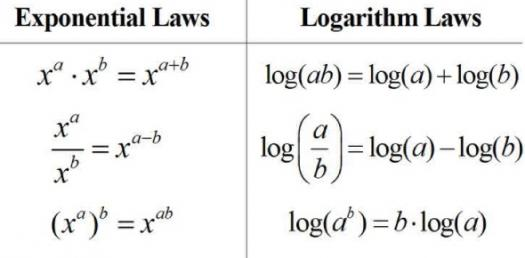# Exponents And Logarithms Quiz 2

13 Questions | Total Attempts: 64SettingsExponents and Logarithms

Related Topics
• 1.
• 2.
Simplify:
• 3.
Simplify:
• 4.
How much more acidic is a substance that has a pH reading of 2 than one that has a pH reading of 7?
• 5.
Approximately how many years will it take you to double your money if you invest it in an account that compounds monthly at an interest rate of 3%?
• 6.
Solve:log5(x - 20) + log5(x) = 3
• 7.
What is the domain of the graph y = log(x + 4)?
• 8.
What is the inverse function of f(x) = log3(x)?Write the equation as y = __________
• 9.
What is inverse of the function y = 10^x?Write as y = ___________
• 10.
Expand:
• 11.
If p = logb(2) and q = logb(7), write in terms of p and q.logb(49/8)
• 12.
Solve: log18 - logx = logx - log2
• 13.
Solve:1/3(lnx) = 4